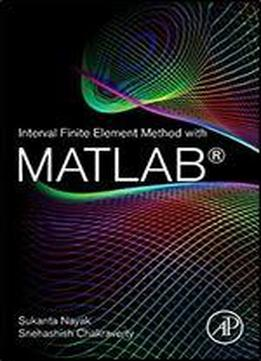# Interval Finite Element Method With MatlabTags: Sukanta Nayak

Interval Finite Element Method with MATLAB provides a thorough introduction to an effective way of investigating problems involving uncertainty using computational modeling. The well-known and versatile Finite Element Method (FEM) is combined with the concept of interval uncertainties to develop the Interval Finite Element Method (IFEM). An interval or stochastic environment in parameters and variables is used in place of crisp ones to make the governing equations interval, thereby allowing modeling of the problem. The concept of interval uncertainties is systematically explained. Several examples are explored with IFEM using MATLAB on topics like spring mass, bar, truss and frame. Provides a systematic approach to understanding the interval uncertainties caused by vague or imprecise data Describes the interval finite element method in detail Gives step-by-step instructions for how to use MATLAB code for IFEM Provides a range of examples of IFEM in use, with accompanying MATLAB codes